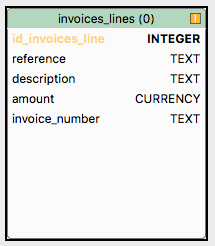# Sql (lite) complex query: find invoices containing two differents products

Hi folks,
I’m looking for a rather complex (for me) sql query.

I have a table with lines of invoices, there can be multiple lines with the same field ‘invoice_number’
I want to select the number of the invoices containing two items “refA” and “refB” in the same invoice

I’m confident this is possible with a single sql query, but can’t figure it !

thanks.

Your table structs are not known to suggest a way. Is it like a table “invoices” and a table “invoice_items” that compose such invoice?

A generic one could be something like SELECT invoice_id FROM invoice_tems WHERE item_code IN (‘refA’, ‘refB’)

as I (tried to ) said, it is only one table, with one record for each line of invoice
and the field 'invoice_number" can be the same for multiple records, telling they belong to the same invoice.I can search for reference=‘refA’ or reference=‘refB’, but how to have only those having the same invoice_number ? I suppose it must be done with subqueries, but can’t figure it.

Maybe this:

SELECT COUNT(*) FROM invoice_lines WHERE invoice_number IN(SELECT invoice_number FROM invoice_lines WHERE reference = ‘refA’ AND invoice_number IN(SELECT invoice_number FROM invoice_lines WHERE reference = ‘refB’))

Edit: invoice_number

1 Like

Assuming the problem is that you want all lines from such invoices, something like this perhaps. (Off the top of my head.)

``````select
*
from
invoice_lines as il1
where
exists (
select * from invoice_lines as il2
where il2.invoice_number = il1.invoice_number
and il2.reference = refA
limit 1
) and exists (
select * from invoice_lines as il2
where il2.invoice_number = il1.invoice_number
and il2.reference = refB
limit 1
)
``````
1 Like

Or this:

``````with
refA as (
select invoice_number from invoice_lines where reference = 'refA'
),
refB as (
select invoice_number from invoice_lines where reference = 'refB'
)
select *
from invoice_lines
where
invoice number in (select invoice_number from refA)
and invoice_number in (select invoice_number from refB)
``````
1 Like

Invoice MUST contain at least one of each:

``````SELECT invnum AS invoice_number
FROM (SELECT MAX(invoice_number) AS invnum,
SUM(IF(reference='A',1,0)) AS quant1,
SUM(IF(reference='B',1,0)) AS quant2
FROM invoices_lines GROUP BY invoice_number) AS sel
WHERE sel.quant1>0 AND sel.quant2>0;
``````

OBS: MySQL dialect

1 Like

setting the first answer from Kem as the solution, because it is the first working.
thanks to all the participants !1 Like

I missed that you were looking for a count of such invoices.

``````with
refA as (
select invoice_number from invoice_lines where reference = 'refA'
),
refB as (
select invoice_number from invoice_lines where reference = 'refB'
)
select count(distinct invoice_number)
from (
(select invoice_number from refA)
intersect
(select invoice_number from refB)
) as t
``````

no I don’t need a count, I just want all the lines .
edit: oups I said “the number of invoices” on the first post. sorry.
so may be David wins after all.

In that case, my second offering is probably more efficient, but glad you got it working.

Clients!

2 Likes

edit: oups I said “the number of invoices” on the first post. sorry.
so may be David wins after all.

Now that I see it on my computer (as opposed to my phone), that’s a nice solution and should be pretty efficient.

yes the second solution you gave seems twice as fast as the first one !